# QuantumClone and QuantumCat

R package available on CRAN or on github

Maintainer: Paul Deveau (paul.deveau at curie.fr)

## Clonal Reconstruction from High-Throughput Sequencing data

QuantumClone is an algorithm that is designed to reconstruct clonal populations (i.e. group of cells with the same genetic background) based on high throughput sequencing data (either whole exome or whole genome) It takes into account information from variants (reads supporting the alternative allele and depth at the position), as well as information from copy number : number of alleles at locus (a normal diploid region would be written as “AB”) Additional information, such as the contamination, is also used.

### Usage

QuantumClone is looking for clones in your samples assuming that there is an evolutionary logic between samples, so you should use data from the same patient for one analysis (either different timepoints, or spatially separated samples, or biological replicates).

#### Input data

QuantumClone requires few informations in the input file:

• Line 1 should be the column titles (Sample | Chr | Start | Alt | Depth ). An additional argument is required if you do not have a FREEC profile associated to your files: the Genotype.

• The first column needs to be the name of your sample

• The Chr column contains the chromosome of variant (e.g. “chr2”)

• Start is the position of the variant

• Alt is the number of reads supporting the variant

• Depth is the depth of coverage at the position of the variant (number of reads mapped at this position)

We show below an example created by the QuantumCat function, and that can be accessed from the data:

    # Example was generated calling:
Input_Example<-QuantumCat(number_of_clones = 4,
number_of_mutations = 100,
ploidy = "AB",depth = 150,
number_of_samples = 2,
contamination = c(0,0))
SampleName Chr Start Depth Alt Genotype
Timepoint_1 1 1 149 67 AB
Timepoint_1 4 2 162 2 AB
Timepoint_1 4 3 132 5 AB
Timepoint_1 4 4 57 1 AB
Timepoint_1 4 5 93 0 AB
Timepoint_1 4 6 95 0 AB

Any additional column will not be taken into account for the analysis

While the input file can be as large as you want, the computation time will exponentially grow with the number of variants to be studied. In order to keep computation time reasonable (from a minute to an hour), a reasonable set of mutation is between 100 to 1000 variants.

#### Analysis

The QuantumClone package is divided in two:

#### Clonal reconstruction

One_step_clustering() has several parameters required (some have default configuration):

  One_step_clustering(SNV_list = Input_Example, FREEC_list = NULL, contamination = c(0,0),
nclone_range = 2:5, clone_priors = NULL, prior_weight = NULL,
Initializations = 1 , preclustering = "Flash",
simulated = FALSE, epsilon = 5 * (10^(-3)),
save_plot = TRUE, ncores = 1,
restrict.to.AB = FALSE, output_directory = NULL)
• SNV_list: list of dataframes. See previous section for description.
• FREEC_list: list of outputs from FREEC (in the same order as the SNV list). See here for added information.
• contamination: Numeric vector giving the fraction of normal cells in each sample. Is linked to the cellularity by contamination = 1 - Cellularity
• nclone_range: number of clones to look for in the samples
• clone_priors: list of vectors giving the position of the clones in each samples (if know from previous analysis)
• prior_weight : fraction of variants belonging to a clone (if known from previous analysis)
• maxit : number of iterations to run per condition. The output will take the maximal maximum likelihood on all iterations.
• preclustering : should kmeans (fpc package) be used to give priors to the alorithm?
• simulated : is the data generated by QuantumCat? It does not change the parameters, but will attribute shapes to different chromosomes in the plots. (see QuantumCat for more information)
• epsilon : stop condition for the EM.
• save_plot : save the 2D plots in a folder with the patient name/output_directory.
• ncores: number of CPUs on which to distribute calculations (used if high number of variants)
• restrict.to.AB : should the clustering be done only on AB regions?
• output_directory : directory in which the plots will be saved (if NULL, will create a directory with the patient name)

The output should look like this: > QC_output$filtered.data[] Chr Start Cellularity Genotype Alt Depth NC NCh alpha id 1 1 0.8993289 AB 67 149 1 2 1 1 4 2 0.0246914 AB 2 162 1 2 1 2 4 3 0.0757576 AB 5 132 1 2 1 3 4 4 0.0350877 AB 1 57 1 2 1 4 4 5 0.0000000 AB 0 93 1 2 1 5 4 6 0.0000000 AB 0 95 1 2 1 6 #### Plots Output from clustering can be represented thanks to the plot_QC_out(), or plot_with_margins_densities (if 1 or two samples) plot_QC_out(QC_output) ## Only one model identified. ## Two samples identified.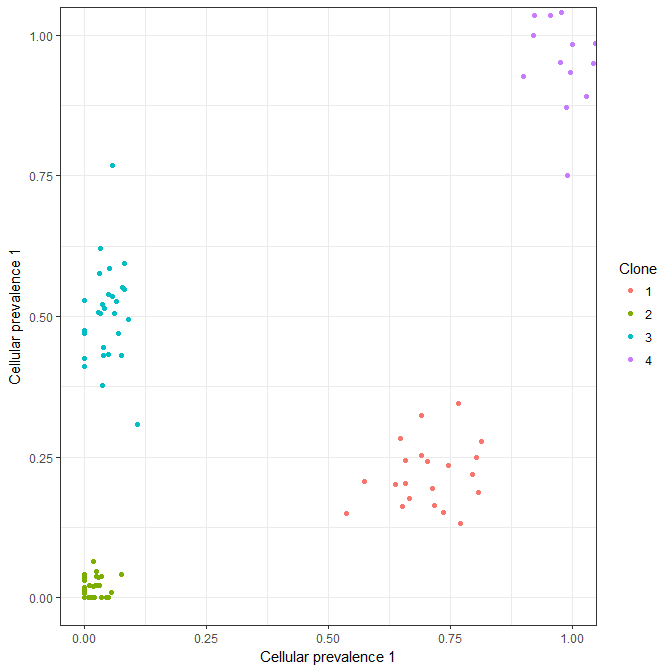If more than one sample (here we reuse sample 1 in the plot, to illustrate plot possibilities, not clustering): > plot_QC_out(QC_output3s, Sample_names=c(“Diag”,“Rel”,“Metastasis”), simulated = FALSE,sample_selected = 1:3)) ## Only one model identified.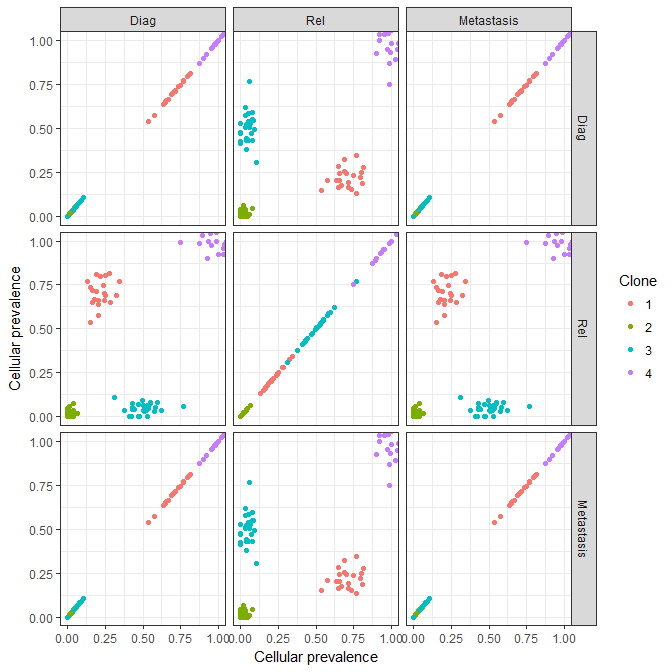plot_with_margins_densities(QC_output)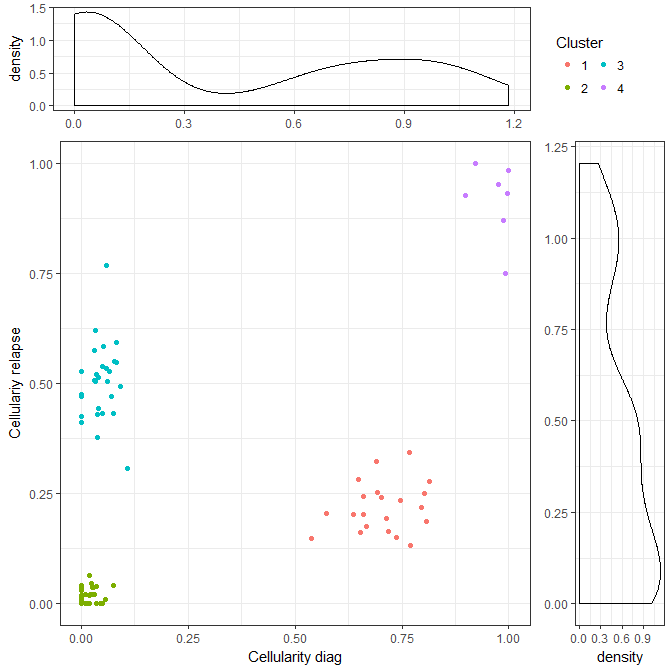For time series, using evolution_plot() is recommanded. It enables the plot of the cellularity of each clone in a single plot, with the width of a line being proportional to the fraction of mutations in the clone. evolution_plot(QC_output,Sample_names = c(“Timepoint_1”,“Timepoint_2”))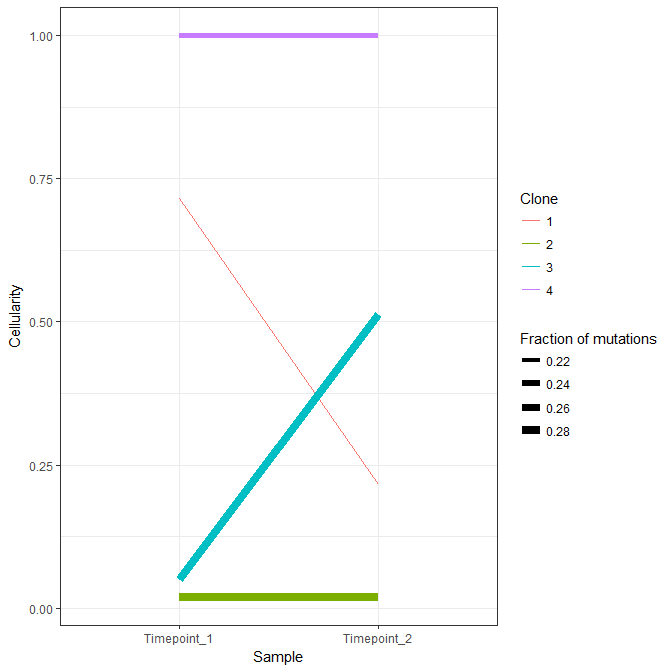#### Recreate phylogenetic tree (when possible) Cellularities<-cbind(QuantumClone::QC_output$EM.output$centers[],QuantumClone::QC_output$EM.output\$centers[])
Tree<-QuantumClone::Tree_generation(Cellularities)

Output of Tree_generation is a list of dataframes and probabilities, as this:

 0 1 0 0 1 0 0 1 1 0 0 0 1 0 0 1 0.714859 0.216637 0 0 0 0 0 0 0 0.0502218 0.51291 0 0 0 0 0 0 0 0.0208175 0.0186199 0 0 1 0 0 1 0 0.285141 0.783363 0 0 0 0 0 0 0 0.234919 0.270453 0 0 0 0 0 0 0 0.694041 0.198018

Each row (i) corresponds to a clone. The last two columns are the cellularity of the clone in the sample. For the other columns (j) there is a 1 if clone j is a progeny of clone (i)

#### Plot all possible phylogenetic trees:

QuantumClone::multiplot_trees(Tree,d = 4)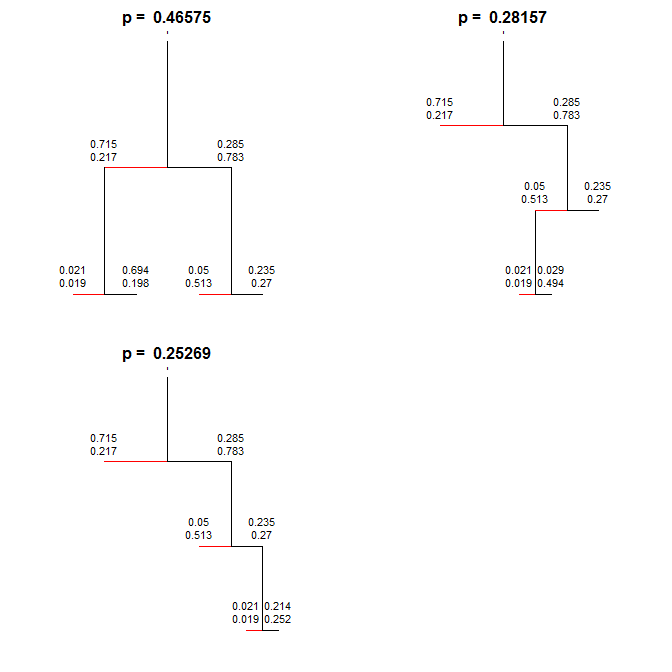#### Clonal simulation

This part is about generating data to test clonal reconstruction algorithms. Its core is the QuantumCat function. It will generate data for a single cancer that can be sequenced multiple times (either spatially separated or different timepoints). It thus assumes that there is an evolutionary history between samples. The “Chr” columns stores the information of the clonal attribution.

QuantumCat(number_of_clones, number_of_mutations, ploidy = 2, depth = 100, number_of_samples = 2, Random_clones = F, contamination = NULL)

• number_of_clones : How many clones should exist in total. For example, 5 clones in 2 samples can be distributed in the following way: 1 specific of sample 1, 1 specific of sample 2 and 3 shared between sample 1 and 2.
• number_of_mutations : How many variants should be used for the clustering. Some algorithms reported that an increase in the number of variants decreased the clustering quality, which does not seem to be the case here. It affects the computing time however.
• ploidy : if numeric, it will generate a poisson distribution with mean the ploidy. Accepted inputs can be “disomic”, “AB”, “AAB”, “A”, etc.
• depth : what is the sequencing depth? Depth of a variant will be generated according to a negative binomial distribution, which characteristics have been generated by fitting to our data from whole genome sequencing.
• number_of_samples = How many samples should be generated?
• Random_clones: if the number of clones should be generated randomly (sampled from 2:5)
• contamination: estimation of the contamination by normal cells

For multiple testings, and calculation of the Normalized Mutual Information (NMI), see Multitest() and statistics_on_Multitest()

### Acknowledgments

Many thanks to the contributors of this work: my supervisors, Elodie for the features improvement and Linux debugging, Matahi for the OSX feedback, and more generally to the U830 & U900 people. This work had been funded by the Ministere de l’Enseignement Superieur de la Recherche (AMX grant).